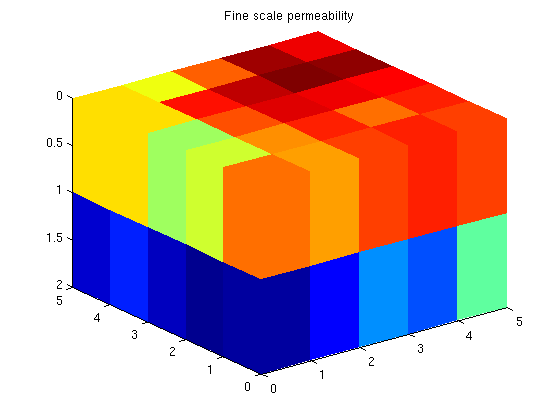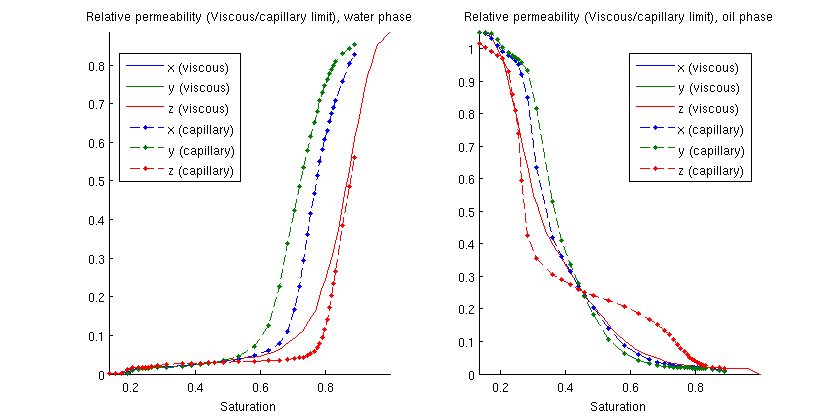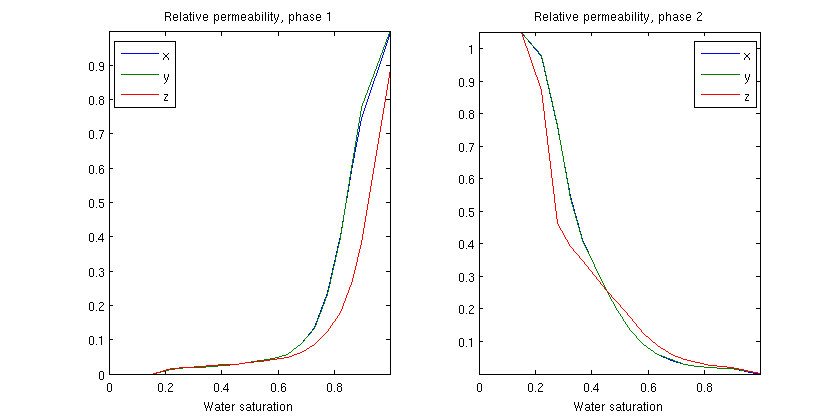#This example demonstrates upscaling of relative permeability on periodic grids. To this end, we upscale a single block sampled from SPE10 using first a standard flow-based method to determine the effective permeability and then finds relative permeability curves based on steady-state upscaling for the capillary and the viscous limits, as well as based on a dynamic simulation.

## Contents

```try
require upscaling spe10
catch %#ok<CTCH>
end
```

## Set up a simple grid with periodic boundaries

Make a grid in which the right boundary wraps around with left boundary, the front with the back, and the bottom with the top. We retain the regular grid for plotting, as plotGrid uses the boundary faces to plot grids: A fully periodic grid has, per definition, no boundary faces.

```G   = cartGrid([5 5 2]);
G   = computeGeometry(G);

% Set faces for wrap-around
bcr{1}=pside([],G,'RIGHT',0);   bcl{1}=pside([],G,'LEFT',0);
bcr{2}=pside([],G,'FRONT',0);   bcl{2}=pside([],G,'BACK',0);
bcr{3}=pside([],G,'BOTTOM',0);  bcl{3}=pside([],G,'TOP',0);

% Make periodic grid.
[Gp, bcp]=makePeriodicGridMulti3d(G, bcl, bcr, {0, 0, 0});
```

## Extract a small subset of SPE10 to upscale.

```x = 51; y = 11; z = 1;

rock = SPE10_rock(x:(x-1+G.cartDims(1)),...
y:(y-1+G.cartDims(2)),...
z:(z-1+G.cartDims(3)));
clf
plotCellData(G, log10(rock.perm(:,1))); view(3); axis tight
title('Fine scale permeability')
```## Do a single-phase periodic upscale

To find the permeability we use a unitary fluid so that the mobility/relperm is equal to the saturation which is equal to one, removing any fluid specific effects. We upscale the permeability using two-point flux approximation for the pressure solver.

```psolver = @(state, Grid, Fluid, BCP, Rock)...
incompTPFA(state, Grid, computeTransGp(G, Grid, Rock),...
Fluid, 'bcp', BCP);
L = max(G.faces.centroids) - min(G.faces.centroids);
fluid_pure = initSingleFluid('mu',1,'rho',1);

warning('off', 'mrst:periodic_bc')
perm2 = upscalePermeabilityPeriodic(Gp, bcp, 1, psolver, fluid_pure, rock, L);
warning('on', 'mrst:periodic_bc')
```

## Load a two-phase fluid for upscaling

The data are synthetic and should not be used for anything but testing. The file 'rocklist.txt' contains a list of included property files in a simple format tabulated on water saturation.

```current_dir = fileparts(mfilename('fullpath'));
fn    = fullfile(current_dir, 'rocklist.txt');

% Print the tabulated values from the first and only file
fprintf('\n');
fprintf(' Sw          | Krw         | Kro         | J-func\n')
fprintf('-------------|-------------|-------------|------------\n')
fprintf(' %+1.4e | %+1.4e | %+1.4e | %+1.4e\n', T{1})
fprintf('\n');

fluid = initSWOFFluidJfunc('mu' , [   10,  100] .* centi*poise     , ...
'rho', [1000, 600] .* kilogram/meter^3, ...
'table', T, ...
'satnum', 1, 'jfunc', true, 'rock', rock, ...
'surf_tens', 10*dyne/(centi*meter));
```
``` Sw          | Krw         | Kro         | J-func
-------------|-------------|-------------|------------
+1.6380e-01 | +2.0870e-01 | +2.4530e-01 | +2.7820e-01
+3.0570e-01 | +3.4470e-01 | +4.4600e-01 | +4.8450e-01
+5.1050e-01 | +5.5230e-01 | +5.9430e-01 | +6.2280e-01
+6.5200e-01 | +7.2460e-01 | +7.6780e-01 | +8.2710e-01
+8.5990e-01 | +8.9540e-01 | +9.2560e-01 | +9.5610e-01
+9.9720e-01 | +0.0000e+00 | +1.7000e-02 | +1.7500e-02
+1.8300e-02 | +1.9000e-02 | +2.0400e-02 | +2.8300e-02
+3.3900e-02 | +3.6000e-02 | +4.1100e-02 | +4.5600e-02
+5.2200e-02 | +5.9400e-02 | +1.0710e-01 | +1.6320e-01
+3.2400e-01 | +4.7220e-01 | +6.3920e-01 | +7.6180e-01
+8.5000e-01 | +8.8560e-01 | +1.0510e+00 | +9.6900e-01
+8.1380e-01 | +6.6210e-01 | +5.3770e-01 | +4.2750e-01
+2.6110e-01 | +2.0970e-01 | +1.8090e-01 | +1.2850e-01
+9.1200e-02 | +7.1900e-02 | +5.7800e-02 | +3.3800e-02
+2.6600e-02 | +2.1200e-02 | +1.9000e-02 | +1.7400e-02
+1.7100e-02 | +1.7000e-02 | +0.0000e+00 | +2.3538e+00
+2.2030e-01 | +1.1690e-01 | +8.8500e-02 | +7.6600e-02
+6.4200e-02 | +4.2000e-03 | -3.0800e-02 | -3.6800e-02
-5.0000e-02 | -6.0900e-02 | -6.7800e-02 | -7.5700e-02
-9.5600e-02 | -1.0690e-01 | -1.2990e-01 | -1.6020e-01
-2.4550e-01 | -3.2290e-01 | -4.6560e-01 | -1.4570e+00

Using rock.perm(:,1) for j-scaling
```

We assume zero capillary forces and do a steady-state upscale using the viscous and capillary limits, respectively. The viscous limit is equal in all directions, while the capillary is not

```[saturations_visc, kr_visc] = ...
upscaleRelpermLimit(G, rock, fluid, 'type', 'fixed', 'limit', 'viscous');
[saturations_cap, kr_cap]   = ...
upscaleRelpermLimit(G, rock, fluid, 'type', 'fixed', 'limit', 'capillary');

% Plot the results
clf;
p = get(gcf,'Position'); set(gcf,'Position',[p(1:2) 840 420]);
ph = {'water', 'oil'};
for i = 1:2
subplot(1,2,i)
hold on
plot(saturations_visc, kr_visc{i});
plot(saturations_cap, kr_cap{i}, '--.');
title(['Relative permeability (Viscous/capillary limit), ' ph{i} ' phase']);
hold off; axis tight
xlabel('Saturation')
legend({'x (viscous)', 'y (viscous)', 'z (viscous)'...
'x (capillary)', 'y (capillary)', 'z (capillary)'}, 'location', 'Best')
end
```In the general case, we initialize the model with a certain fraction of water and simulate the dynamic behaviour due to a linear pressure drop in until steady-state is reached. This way, we can tabulate relative permeability versus average water saturation. Here, we use ~20 data points. As the default option is to use a pressure drop in x-direction, the x-values are significantly different from the y/z values which are similar, but not equal.

```saturations = 0:0.05:1;
dp_scale=1e-3;

% Ignore warnings from the implicit solver as the solution is driven to
% steady state. It is natural that some steps fail during this process.
warning('off', 'implicitTransport:failure')
[sat_vec, kr, perm, krK] = upscaleRelperm(G, rock, fluid, dp_scale, saturations, 'periodic', false);
warning('on', 'implicitTransport:failure')

% Plot the resulting relative permeability
for i = 1:2
subplot(1,2,i)
plot(sat_vec, kr{i});
title(['Relative permeability, phase ' num2str(i)]);
axis tight
xlabel('Water saturation')
legend({'x', 'y', 'z'}, 'location', 'Best')
end
```Published June 4, 2013